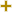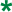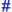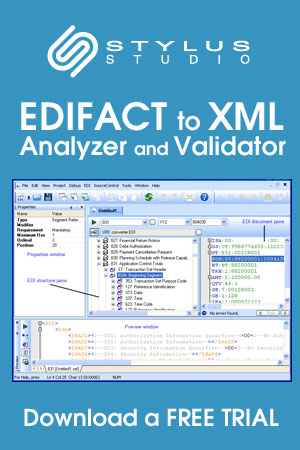# Date/time/period format qualifierUN/CEFACT Revision 1997A Code ListRepr:an..3
Desc:Specification of the representation of a date, a date and time or of a period.
This table also occurs in the following versions of this standard:
D00A, D00B, D01A, D01B, D01C, D02A, D02B, D03A, D03B, D04A, D04B, D93A, D94A, D94B, D95A, D95B, D96A, D96B, D97A, D97B, D98A, D98B, D99A, D99B

 2 DDMMYY Calendar date: D = Day; M = Month; Y = Year. 3 MMDDYY Calendar date: M = Month; D = Day; Y = Year. 101 YYMMDD Calendar date: Y = Year; M = Month; D = Day. 102 CCYYMMDD Calendar date: C = Century ; Y = Year ; M = Month ; D = Day. 103 YYWWD Calendar week day: Y = Year ; W = Week ; D = Day Week number 01 is always first week of January Day number 1 is always Monday. 105 YYDDD Calendar day: Y = Year ; D = Day January the first = Day 001 Always start numbering the days of the year from January 1st through December 31st. 106 MMDD Day of a month: M = Month; D = Day. 107 DDD Day's number within a specific year: D = Day. 108 WW Week's number within a specific year: W = Week. 109 MM Month's number within a specific year: M = Month. 110 DD Day's number within is a specific month: D = Day. 201 YYMMDDHHMM Calendar date including time without seconds: Y = Year; M = Month; D = Day; H = Hour; M = Minute. 202 YYMMDDHHMMSS Calendar date including time with seconds: Y = Year; M = Month; D = Day; H = Hour; m = Minutes = Seconds. 203 CCYYMMDDHHMM Calendar date including time with minutes: C=Century; Y=Year; M=Month; D=Day; H=Hour; M=Minutes. 204 CCYYMMDDHHMMSS Calendar date including time with seconds: C=Century;Y=Year; M=Month;D=Day;H=Hour;M=Minute;S=Second. 301 YYMMDDHHMMZZZ See 201 + Z = Time zone. 302 YYMMDDHHMMSSZZZ See 202 + Z = Time zone. 303 CCYYMMDDHHMMZZZ See 203 plus Z=Time zone. 304 CCYYMMDDHHMMSSZZZ See 204 plus Z=Time zone. 305 MMDDHHMM Month, day, hours, minutes; M = Month; D = Day; H = Hour; M = Minute. 306 DDHHMM Day, hours, minutes; D = Day; H = Hour; M = Minute. 401 HHMM Time without seconds: H = Hour; m = Minute. 402 HHMMSS Time with seconds: H = Hour; m = Minute; s = Seconds. 404 HHMMSSZZZ See 402 plus Z=Time zone. 405 MMMMSS Time without hours: m=minutes, s=seconds. 501 HHMMHHMM Time span without seconds: H = Hour; m = Minute;. 502 HHMMSS-HHMMSS Format of period to be given without hyphen. 503 HHMMSSZZZ-HHMMSSZZZ Format of period to be given without hyphen. 600 CC Century. 601 YY Calendar year: Y = Year. 602 CCYY Calendar year including century: C = Century; Y = Year. 603 YYS Semester in a calendar year: Y = Year; S = Semester. 604 CCYYS Semester in a calendar year: C = Century; Y = Year; S = Semester. 608 CCYYQ Quarter in a calendar year: C = Century; Y = Year; Q = Quarter. 609 YYMM Month within a calendar year: Y = Year; M = Month. 610 CCYYMM Month within a calendar year: CC = Century; Y = Year; M = Month. 613 YYMMA Format of period to be given without hyphen (A = ten days period). 614 CCYYMMA Format of period to be given without hyphen (A = ten days period). 615 YYWW Week within a calendar year: Y = Year; W = Week 1st week of January = week 01. 616 CCYYWW Week within a calendar year: CC = Century; Y = Year; W = Week (1st week of January = week 01). 701 YY-YY Format of period to be given in actual message without hyphen. 702 CCYY-CCYY Format of period to be given in actual message without hyphen. 703 YYS-YYS Format of period to be given without hyphen. 704 CCYYS-CCYYS Format of period to be given in actual message without hyphen. 705 YYPYYP Format of period to be given without hyphen (P = period of 4 months). 706 CCYYP-CCYYP Format of period to be given without hyphen (P = period of 4 months). 707 YYQ-YYQ Format of period to be given without hyphen. 708 CCYYQ-CCYYQ Format of period to be given in actual message without hyphen. 709 YYMM-YYMM Format of period to be given in actual message without hyphen. 710 CCYYMM-CCYYMM Format of period to be given in actual message without hyphen. 711 CCYYMMDD-CCYYMMDD Format of period to be given in actual message without hyphen. 713 YYMMDDHHMM-YYMMDDHHMM Format of period to be given in actual message without hyphen. 715 YYWW-YYWW Format of period to be given in actual message without hyphen. 716 CCYYWW-CCYYWW Format of period to be given without hyphen. 717 YYMMDD-YYMMDD Format of period to be given in actual message without hyphen. 718 CCYYMMDD-CCYYMMDD Format of period to be given without hyphen. 801 Year To indicate a quantity of years. 802 Month To indicate a quantity of months. 803 Week To indicate a quantity of weeks. 804 Day To indicate a quantity of days. 805 Hour To indicate a quantity of hours. 806 Minute To indicate a quantity of minutes. 807 Second To indicate a quantity of seconds. 808 Semester To indicate a quantity of semesters (six months). 809 Four months period To indicate a quantity of four months periods. 810 Trimester To indicate a quantity of trimesters (three months). 811 Half month To indicate a quantity of half months. 812 Ten days To indicate a quantity of ten days periods. 813 Day of the week Numeric representation of the day (Monday = 1). 814 Working days Number of working days.

Change indicators
plus signAn addition.
asteriskAddition/substraction/change to a code entry for a particular data element.
hash or pound signChanges to names.
vertical barChanges to text for descriptions, notes and functions.
minus signA deletion.
letter XMarked for deletion.

Usage indicatorsUsed in batch messages only.Common usage in both batch and interactive messages.Used in interactive messages only.